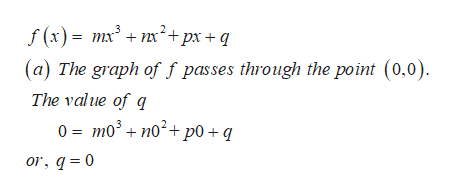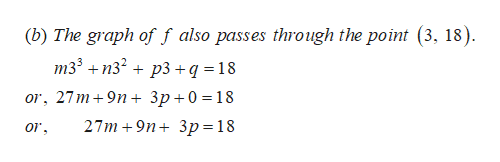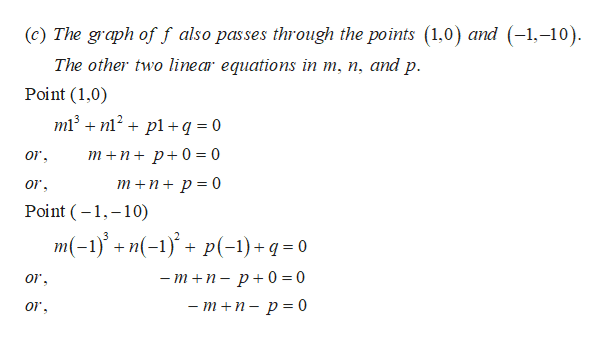# The function f is given by f(x) = mx3+ nx2+ px + q, where m, n, p, q are integers.The graph of f passes through the point (0, 0).(a) Write down the value of q.The graph of f also passes through the point (3, 18).(b) Show that 27m+ 9n + 3p =18.The graph of f also passes through the points (1, 0) and (–1, –10).(c) Write down the other two linear equations in m, n, and p.(d) Find the function in standard form(e) The function f can also be written f (x) = x (x −1)(r x − s) where r and s areintegers. Find r and s.

Question
40 views

The function f is given by f(x) = mx3
+ nx2
+ px + q, where m, n, p, q are integers.
The graph of f passes through the point (0, 0).
(a) Write down the value of q.
The graph of f also passes through the point (3, 18).
(b) Show that 27m+ 9n + 3p =18.
The graph of f also passes through the points (1, 0) and (–1, –10).
(c) Write down the other two linear equations in m, n, and p.
(d) Find the function in standard form
(e) The function f can also be written f (x) = x (x −1)(r x − s) where r and s are
integers. Find r and s.

check_circle

Step 1

(a) the value of q.help_outlineImage Transcriptionclosef(x)= mx +nx +px + q (a) The graph of f passes through the point (0,0) The value of q 0 =mo3 n0+ p0 + q or, q 0 fullscreen
Step 2

(b)help_outlineImage Transcriptionclose(b) The graph of f also passes through the point (3, 18) m33 n32 p3 q = 18 or, 27 m + 9n + Зр+0 %3D18 27m + 9n+ 3р%3D18 or fullscreen
Step 3help_outlineImage Transcriptionclose(c) The graph off also passes through the points (1,0) and (-1.-10). The other two linear equations in m, n, and p. Point (1,0) m13 n12pl +q = 0 т+n+ p+0%3D0 т +n+ p%3 0 or Point 1 10) m(-1)n(-p-1)+q= 0 3 — т +п- р+ 0%3D0 or — т +п- р%3D0 or fullscreen

### Want to see the full answer?

See Solution

#### Want to see this answer and more?

Solutions are written by subject experts who are available 24/7. Questions are typically answered within 1 hour.*

See Solution
*Response times may vary by subject and question.
Tagged in

### Other